# Minimal ideal

(diff) ← Older revision | Latest revision (diff) | Newer revision → (diff)

A minimal element of the partially ordered set of ideals of a given type of some algebraic system. Since the ordering on the set of ideals is defined by the inclusion relation, a minimal ideal is an ideal not containing ideals of the same type different from itself. For multi-operator groups (in particular, rings) and for lattices, in contrast to semi-groups, it is always assumed that the partially ordered set of ideals does not contain the zero ideal. If no class of ideals is specifically mentioned, a minimal ideal is taken to be minimal in the set of all (non-zero) two-sided ideals.

A minimal two-sided ideal, if one exists, in a semi-groupis unique and is the smallest two-sided ideal: it is called the kernel of the semi-group. Not every semi-group has a kernel (for example, an infinite monogenic semi-group) but, for example, the kernel exists in any finite semi-group. The kernel is an ideally-simple semi-group (see Simple semi-group). If the kernel of a semi-groupis a group, thenis called a homogroup. A semi-groupis a homogroup if and only if there is an elementinwhich is divisible on the left and right by any element of(that is,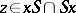for any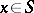); in this case the kernel consists of all such elements. For example, every finite commutative semi-group is a homogroup.

If a semi-grouphas a minimal left ideal, then for anythe product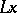is also a minimal left ideal, moreover, every minimal left ideal can be obtained in this way. Every minimal left ideal is a left simple semi-group. In a semi-group with minimal left ideals every left ideal contains a minimal left ideal, and the union of all minimal left ideals (which are pairwise disjoint) is the kernel of the semi-group. If a semi-grouphas a minimal left idealand a minimal right ideal, thenis a subgroup inand,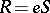, whereis the identity of this subgroup; the product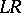coincides with the kernel ofand is, in this case, a completely-simple semi-group.

For semi-groups with a zero, the interest is in the consideration of non-zero ideals, and a minimal element in the corresponding partially ordered set of ideals is called a-minimal (left, right, two-sided) ideal. The properties of-minimal ideals are in many ways similar to the properties of minimal ideals, with some natural restrictions. For example, a-minimal two-sided ideal is not necessarily unique and need not be a-simple semi-group; it may be a semi-group with zero multiplication (see Nilpotent semi-group). The union of all-minimal left ideals (respectively,-minimal right ideals) is a semi-group with zero, called its left (respectively, right) socle (by definition, the socle is equal to zero if there are no corresponding-minimal ideals in the semi-group). A semi-group coincides with its left and right socles if and only if it is an-direct union of completely-simple semi-groups and semi-groups with zero multiplication.

The consideration of minimal ideals and-minimal ideals plays an essential role in the structure theory of a number of important classes of semi-groups (see, for example, Completely-simple semi-group; Regular semi-group, and also , §§ 2.5, 2.7, Chapt. 6, §§ 7.7, 8.2, 8.3; , Chapt. V).

L.N. Shevrin

Rings (like semi-groups) need not have minimal ideals (the simplest example is the ring of integers) and a minimal ideal in a ring, if it exists, need not be unique. The sum of all (left, right, two-sided) minimal ideals in a ring is called the (left, right, two-sided) socle of the ring. An Artinian ring, obviously, has a non-zero socle. The presence of minimal ideals in a primitive ring makes it close to a matrix ring in the following sense: A primitive ring with a non-zero socle is isomorphic to a dense subring of the ring of all linear transformations of some vector space over a skew-field, containing all transformations of finite rank .

V.E. Govorov

References for the above sections are given below.